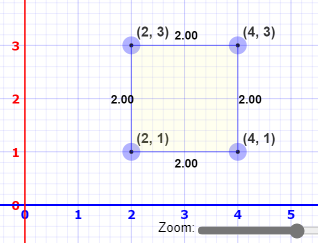# Bounding Box coordinate format

I’m confused by how bounding boxes are defined.

in detection bbox, a coordinate is formatted as `((xmin, ymin), (xmax,ymax))`:

`(xmin, ymin)` — indicates top-left corner of bbox.
`(xmax,ymax)` — indicates bottom-right corner of bbox.

But from plane of a sample box:Coordinate of the box is` ((2, 3), (4,1))`, from this the format should be:

`((xmin, ymax), (xmax,ymin))`

What is the logic in the detection bbox format of `((xmin, ymin), (xmax,ymax))`?

That is true in the context of standard mathematical expression.

However, image processing packages such as PIL or cv2 determine the top left corner to be the origin. That means the top left corner would be (x,y)=(0,0). As it gets further right-down, it travels to the positive direction. Hence the given coordinate.